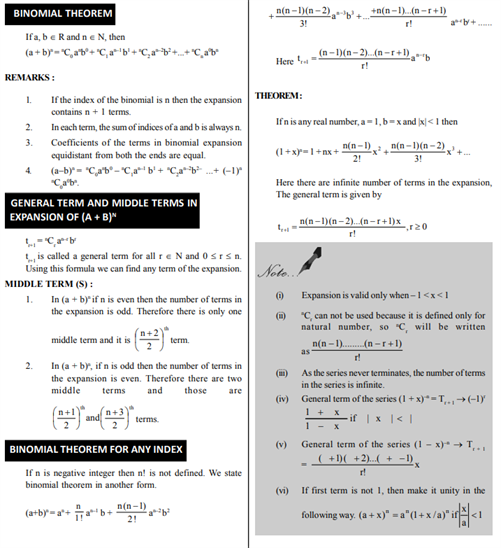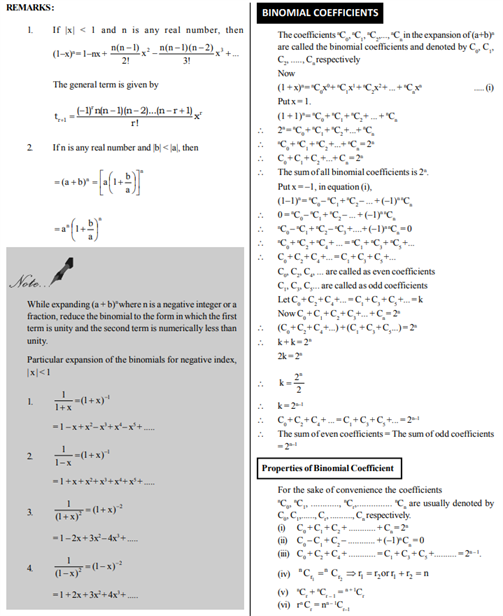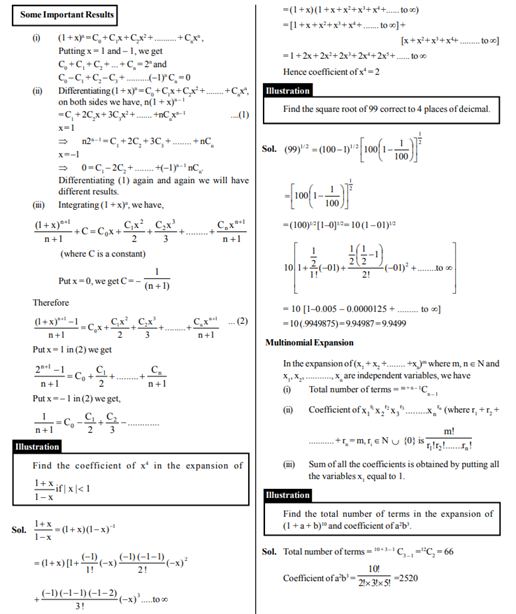# Revision Notes For CBSE Class 11 Maths Chapter 8 Binomial Theorem

Class 11 Maths Revision Notes Chapter 8 Binomial Theorem consists of all the important theories and explanations related to this chapter. Students will be benefiting from this notes when it comes to getting some clarity with respect to this chapter. We are aware of the definition of Binomial Theorem:

• The binomial theorem is used to expand binomial expressions (a + b) raised to any given power without direct multiplication. For example: (a + b)3 = a3 + 3 a2b + 3 ab2 + b3 Starting with the first term and progressing to the last, the exponent of a decrease by one while the exponent of b increases by one, and the sum of the exponents of a and b in each term is n. The binomial coefficients form a pattern of 1 3 3 1. The coefficients correspond with the rows of Pascal’s triangle and are determined by a formula involving factorials.

These observations form the basis of the binomial theorem in its expanded form: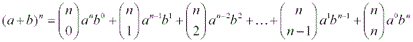Using sigma notation and factorials, the binomial theorem becomes: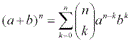where the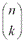notation is a combination such as nCk (read “n choose k”):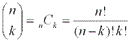The notes are carefully designed by our subject experts and they are arranged systematically for your convenience. These notes can be referred every time when they face trouble while practicing problems. Having these notes handy will prove to be beneficial. Students will get to learn the binomial theorem of any index and binomial coefficients etc.

Find the class 11 maths revision notes Chapter 8 Binomial Theorem below.